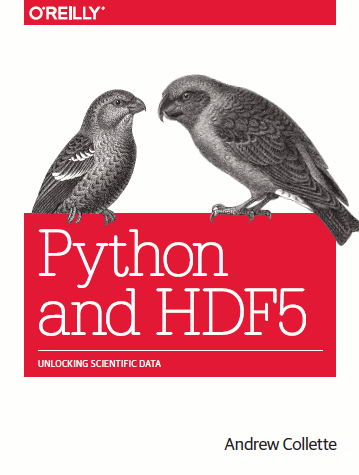# Sparse matrices with h5py

Published: December 12, 2017. Updated: February 20, 2018.HDF5 is an established language-independent, cross-platform binary dataformat, allowing fast and partial loading of data from disk into memory. h5py is the established Python API for interacting with HDF5 files. However, neither h5py nor the pytables high-level interface of h5py provide support for sparse matrices.

Within the single-cell genomics community, 10x Genomics and Scanpy adapted the CSR/CSC/Yale for static HDF5 storage. loompy suggested to dynamically load matrices using the COO format. However, no conventions on how different API's would recognize the stored matrices have been established.

Here, I suggest to adapt the h5sparse convention intoduced by Appier, Inc.. The idea is to mark a HDF5 group that stores a sparse matrix using two attributes

• the storage format of the sparse matrix: h5sparse_format
• the shape of the sparse matrix: h5sparse_shape

These are sufficient to recoqnize a group as a sparse matrix of a specific format and shape.

We implemented this convention in AnnData's submodule anndata.h5py, a thin layer for h5py that offers all functionality of h5py and is able to handle HDF5 files that store both dense and sparse data in different formats. The original h5sparse, by contrast, follows different design principles and only allows to deal with HDF5 files that solely contain sparse data with limited indexing options.

Note 1: As Scanpy is based on anndata, it adopts the convention since version 0.4

Note 2: In the future, anndata's support for HDF5 based storage of dictionaries and categorical data might also be integrated into anndata.h5py.

import numpy as np
from scipy.sparse import csr_matrix, csc_matrix
import anndata
from anndata import h5py, logging
print(anndata.__version__)

0.5.3

!rm test.h5


## Indexing sparse matrices on disk as in memory

X_array = np.array(
[[0, 1, 0],
[0, 0, 2],
[0, 0, 0],
[3, 4, 0]])
# make this a sparse matrix
X = csr_matrix(X_array)

X

<4x3 sparse matrix of type '<class 'numpy.int64'>'
with 4 stored elements in Compressed Sparse Row format>


Show it's entries.

print(X)

  (0, 1)    1
(1, 2)    2
(3, 0)    3
(3, 1)    4

X[:, 1:3].toarray()

array([[1, 0],
[0, 2],
[0, 0],
[4, 0]])


Now, let's open a file and create an h5py.SparseDataset.

f = h5py.File('./test.h5')

f.create_dataset('X', data=X)

<HDF5 sparse dataset: format 'csr', shape (4, 3), type '<i8'>

f['X'][:, 1:3].toarray()

array([[1, 0],
[0, 2],
[0, 0],
[4, 0]])

f['X'][np.array([True, False, False, True])].toarray()

array([[0, 1, 0],
[3, 4, 0]], dtype=int64)


Set an element that preserves the sparsity structure.

f['X'][0, 1] = 9

f['X'][:].toarray()

array([[0, 9, 0],
[0, 0, 2],
[0, 0, 0],
[3, 4, 0]])


Set a chunk that preserves the sparsity structure.

f['X'][0:2] = 2*f['X'][0:2]

f['X'][:].toarray()

array([[ 0, 18,  0],
[ 0,  0,  4],
[ 0,  0,  0],
[ 3,  4,  0]])


Append.

f['X'].append(2*f['X'][0:2])

f['X'][:].toarray()

array([[ 0, 18,  0],
[ 0,  0,  4],
[ 0,  0,  0],
[ 3,  4,  0],
[ 0, 36,  0],
[ 0,  0,  8]])


Just check that we can do the same with the array.

f.create_dataset('Y', data=X_array)

<HDF5 dataset "Y": shape (4, 3), type "<i8">

f['Y'][:]

array([[0, 1, 0],
[0, 0, 2],
[0, 0, 0],
[3, 4, 0]])

f.close()


Looking into the file reveals the following structure.

!h5ls -r './test.h5'

/                        Group
/X                       Group
/X/data                  Dataset {6/Inf}
/X/indices               Dataset {6/Inf}
/X/indptr                Dataset {7/Inf}
/Y                       Dataset {4, 3}


The group that stores the sparse matrix is marked by the two attributes h5sparse_format and h5sparse_shape:

!h5ls -v './test.h5'

Opened "./test.h5" with sec2 driver.
X                        Group
Attribute: h5sparse_format scalar
Type:      variable-length null-terminated UTF-8 string
Data:  "csr"
Attribute: h5sparse_shape {2}
Type:      native long
Data:  6, 3
Location:  1:800
Y                        Dataset {4/4, 3/3}
Location:  1:15720
Storage:   96 logical bytes, 96 allocated bytes, 100.00% utilization
Type:      native long


## Within an AnnData object

Initializing an AnnData object with a sparse matrix in memory mode.

from anndata import AnnData, read_h5ad

adata = AnnData(X)


We could now transition to backed mode by setting the .filename attribute.

Instead, let us write the object as an "h5ad"-formatted HDF5 file and open it in backed mode.

adata.write('./test.h5ad')

adata = read_h5ad('./test.h5ad', backed='r+')

adata

AnnData object with n_obs × n_vars = 4 × 3 backed at './test.h5'

adata.X

<HDF5 sparse dataset: format 'csr', shape (4, 3), type '<f4'>

adata.X[0:2] = np.log1p(adata.X[0:2])

adata.X[:].toarray()

array([[0.       , 0.6931472, 0.       ],
[0.       , 0.       , 1.0986123],
[0.       , 0.       , 0.       ],
[3.       , 4.       , 0.       ]], dtype=float32)


Changing from csr to csc of the file.

adata.X

<HDF5 sparse dataset: format 'csr', shape (4, 3), type '<f4'>

adata.X = csc_matrix(X_array)

adata.X

<HDF5 sparse dataset: format 'csc', shape (4, 3), type '<i8'>


## Memory profiling

Let us perform some memory profiling to see whether we really gained something.

logging.print_memory_usage()

Memory usage: current 0.07 GB, difference +0.07 GB

X = csr_matrix(np.ones((10000, 10000)))


This is a really boring, really large matrix filled with $10^8$ ones.

X

<10000x10000 sparse matrix of type '<class 'numpy.float64'>'
with 100000000 stored elements in Compressed Sparse Row format>


It takes about 1.12 GB in memory.

logging.print_memory_usage()

Memory usage: current 1.19 GB, difference +1.12 GB

f = h5py.File('./test2.h5')

f.create_dataset('X', data=X)

<HDF5 sparse dataset: format 'csr', shape (10000, 10000), type '<f8'>

logging.print_memory_usage()

Memory usage: current 1.19 GB, difference +0.00 GB

f.close()


Make sure the memory is actually freed by restarting the notebook, let's start over.

f = h5py.File('./test1.h5')

logging.print_memory_usage()

Memory usage: current 0.07 GB, difference +0.07 GB

f['X'][1:5]

<4x10000 sparse matrix of type '<class 'numpy.float64'>'
with 40000 stored elements in Compressed Sparse Row format>

logging.print_memory_usage()

Memory usage: current 0.07 GB, difference +0.00 GB

f['X'][[1, 5, 10, 13]]

<4x10000 sparse matrix of type '<class 'numpy.float64'>'
with 40000 stored elements in Compressed Sparse Row format>

logging.print_memory_usage()

Memory usage: current 0.07 GB, difference +0.00 GB


Only when loading the full object into memory, we again observe an 1.12 GB increase.

f['X'][:]

<10000x10000 sparse matrix of type '<class 'numpy.float64'>'
with 100000000 stored elements in Compressed Sparse Row format>

logging.print_memory_usage()

Memory usage: current 1.19 GB, difference +1.12 GB